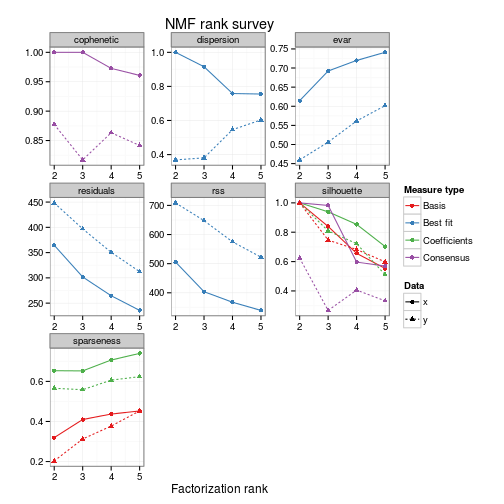# Estimate Rank for NMF Models

## Description

A critical parameter in NMF algorithms is the factorization rank `r`. It defines the number of basis effects used to approximate the target matrix. Function `nmfEstimateRank` helps in choosing an optimal rank by implementing simple approaches proposed in the literature.

In the plot generated by `plot.NMF.rank`, each curve represents a summary measure over the range of ranks in the survey. The colours correspond to the type of data to which the measure is related: coefficient matrix, basis component matrix, best fit, or consensus matrix.

## Usage

```nmfEstimateRank(x, range, method = nmf.getOption("default.algorithm"), nrun = 30,
model = NULL, ..., verbose = FALSE, stop = FALSE)

S3 (NMF.rank)
`plot`(x, y = NULL, what = c("all", "cophenetic", "rss", "residuals", "dispersion",
"evar", "sparseness", "sparseness.basis", "sparseness.coef", "silhouette", "silhouette.coef",
"silhouette.basis", "silhouette.consensus"), na.rm = FALSE, xname = "x", yname = "y",
xlab = "Factorization rank", ylab = "", main = "NMF rank survey", ...)```

## Arguments

x
For `nmfEstimateRank` a target object to be estimated, in one of the format accepted by interface `nmf`. For `plot.NMF.rank` an object of class `NMF.rank` as returned by function `nmfEstimateRank`.
range
a `numeric` vector containing the ranks of factorization to try. Note that duplicates are removed and values are sorted in increasing order. The results are notably returned in this order.
method
A single NMF algorithm, in one of the format accepted by the function `nmf`.
nrun
a `numeric` giving the number of run to perform for each value in `range`.
model
model specification passed to each `nmf` call. In particular, when `x` is a formula, it is passed to argument `data` of `nmfModel` to determine the target matrix -- and fixed terms.
verbose
toggle verbosity. This parameter only affects the verbosity of the outer loop over the values in `range`. To print verbose (resp. debug) messages from each NMF run, one can use `.options='v'` (resp. `.options='d'`) that will be passed to the function `nmf`.
stop
logical flag for running the estimation process with fault tolerance. When `TRUE`, the whole execution will stop if any error is raised. When `FALSE` (default), the runs that raise an error will be skipped, and the execution will carry on. The summary measures for the runs with errors are set to NA values, and a warning is thrown.
...
For `nmfEstimateRank`, these are extra parameters passed to interface `nmf`. Note that the same parameters are used for each value of the rank. See `nmf`. For `plot.NMF.rank`, these are extra graphical parameter passed to the standard function `plot`. See `plot`.
y
reference object of class `NMF.rank`, as returned by function `nmfEstimateRank`. The measures contained in `y` are used and plotted as a reference. It is typically used to plot results obtained from randomized data. The associated curves are drawn in red (and pink), while those from `x` are drawn in blue (and green).
what
a `character` vector whose elements partially match one of the following item, which correspond to the measures computed by `summary` on each -- multi-run -- NMF result: ‘all’, ‘cophenetic’, ‘rss’, ‘residuals’, ‘dispersion’, ‘evar’, ‘silhouette’ (and more specific *.coef, *.basis, *.consensus), ‘sparseness’ (and more specific *.coef, *.basis). It specifies which measure must be plotted (`what='all'` plots all the measures).
na.rm
single logical that specifies if the rank for which the measures are NA values should be removed from the graph or not (default to `FALSE`). This is useful when plotting results which include NAs due to error during the estimation process. See argument `stop` for `nmfEstimateRank`.
xname,yname
legend labels for the curves corresponding to measures from `x` and `y` respectively
xlab
x-axis label
ylab
y-axis label
main
main title

## Value

`nmfEstimateRank` returns a S3 object (i.e. a list) of class `NMF.rank` with the following elements:

measures a `data.frame` containing the quality measures for each rank of factorizations in `range`. Each row corresponds to a measure, each column to a rank. consensus a `list` of consensus matrices, indexed by the rank of factorization (as a character string). fit a `list` of the fits, indexed by the rank of factorization (as a character string).

## Details

Note that from version 0.7, one can equivalently call the function `nmf` with a range of ranks.

Given a NMF algorithm and the target matrix, a common way of estimating `r` is to try different values, compute some quality measures of the results, and choose the best value according to this quality criteria. See Brunet et al. (2004) and Hutchins et al. (2008).

The function `nmfEstimateRank` allows to perform this estimation procedure. It performs multiple NMF runs for a range of rank of factorization and, for each, returns a set of quality measures together with the associated consensus matrix.

In order to avoid overfitting, it is recommended to run the same procedure on randomized data. The results on the original and the randomised data may be plotted on the same plots, using argument `y`.

## References

Brunet J, Tamayo P, Golub TR and Mesirov JP (2004). "Metagenes and molecular pattern discovery using matrix factorization." _Proceedings of the National Academy of Sciences of the United States of America_, *101*(12), pp. 4164-9. ISSN 0027-8424, , .

Hutchins LN, Murphy SM, Singh P and Graber JH (2008). "Position-dependent motif characterization using non-negative matrix factorization." _Bioinformatics (Oxford, England)_, *24*(23), pp. 2684-90. ISSN 1367-4811, , .

## Examples

``````
if( !isCHECK() ){

set.seed(123456)
n <- 50; r <- 3; m <- 20
V <- syntheticNMF(n, r, m)

# Use a seed that will be set before each first run
res <- nmfEstimateRank(V, seq(2,5), method='brunet', nrun=10, seed=123456)
# or equivalently
res <- nmf(V, seq(2,5), method='brunet', nrun=10, seed=123456)

# plot all the measures
plot(res)
# or only one: e.g. the cophenetic correlation coefficient
plot(res, 'cophenetic')

# run same estimation on randomized data
rV <- randomize(V)
rand <- nmfEstimateRank(rV, seq(2,5), method='brunet', nrun=10, seed=123456)
plot(res, rand)

}
````````````
``````